# AP Physics 1 Question 324: Answer and Explanation

### Test Information

Question: 324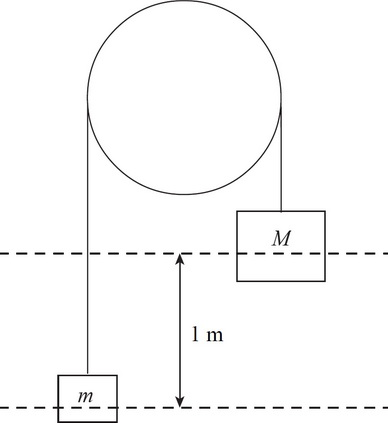2. The pulley above is both frictionless and massless, and the blocks are initially at rest. How long will it take for the two blocks to reach an equal height if block M of mass 15 kg starts 1 m above block m of mass 5 kg?

• A.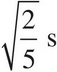• B.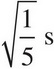• C.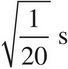• D.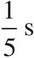Using Newton's Second Law and taking up as the positive direction, you get FNet = ma = T - mg for the first block and FNet = M(-a) = T - Mg for the second block. Subtracting the second equation from the first gives ma + Ma = Mg - mg. Solving for a then gives a =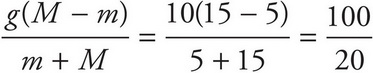= 5 m/s2.
From there, the problem is simply a situation of uniform accelerated motion. The blocks need to move a distance of d = 0.5 m each, the starting speed is 0 m/s, and the acceleration is 5 m/s2. Using d = v0t +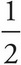at2 gives (0.5) = (0)t +(5)t2, so t =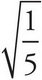s.